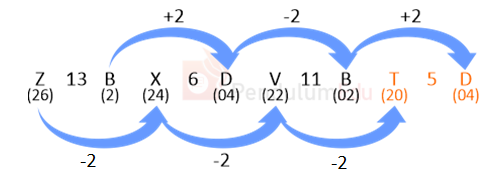Question of The Day10-06-2021

Choose the given alternative that will replace “?” question mark.

Z13B, X6D, V11B, ?

Correct Answer : a ) T5D

Explanation :

According to the question

Let us assume A is numbered as 1, B as 2 and so on till Z being numbered as 26

Z13B, X6D, V11B, ?

The common logic applied here is

We get the number at the middle by dividing the place value of 1st alphabets to the 3rd alphabets$$26 \over 2$$=13

$$24 \over 4$$=6

$$22 \over 2$$=11

Similarly

$$20 \over 4$$=5

Thus, T5D will replace “?”

Hence, (a) is the correct answer.

Questions related to Reasoning are asked in various competitive exams like State PCS, UPSC CDS, SSC CGL, SSC CHSL, SSC CPO, RRB NTPC, UP SI, IB ACIO, etc.

Read Daily Current Affairs, Banking Awareness, Hindi Current Affairs, Word of the Day, Study Material for GK, Study Material for Quant and attempt free mock tests at PendulumEdu and boost your preparation for the actual exam.

Also, Attempt Daily Current Affairs Quizzes, Banking Awareness Quizzes and other exam related Quizzes.0